JEE  >  Chemistry Test 1 - Mole Concept, Atomic Structure, Bonding, Periodic Properties, General Organic Compounds

# Chemistry Test 1 - Mole Concept, Atomic Structure, Bonding, Periodic Properties, General Organic Compounds

Test Description

## 25 Questions MCQ Test JEE Main & Advanced Mock Test Series | Chemistry Test 1 - Mole Concept, Atomic Structure, Bonding, Periodic Properties, General Organic Compounds

Chemistry Test 1 - Mole Concept, Atomic Structure, Bonding, Periodic Properties, General Organic Compounds for JEE 2023 is part of JEE Main & Advanced Mock Test Series preparation. The Chemistry Test 1 - Mole Concept, Atomic Structure, Bonding, Periodic Properties, General Organic Compounds questions and answers have been prepared according to the JEE exam syllabus.The Chemistry Test 1 - Mole Concept, Atomic Structure, Bonding, Periodic Properties, General Organic Compounds MCQs are made for JEE 2023 Exam. Find important definitions, questions, notes, meanings, examples, exercises, MCQs and online tests for Chemistry Test 1 - Mole Concept, Atomic Structure, Bonding, Periodic Properties, General Organic Compounds below.
Solutions of Chemistry Test 1 - Mole Concept, Atomic Structure, Bonding, Periodic Properties, General Organic Compounds questions in English are available as part of our JEE Main & Advanced Mock Test Series for JEE & Chemistry Test 1 - Mole Concept, Atomic Structure, Bonding, Periodic Properties, General Organic Compounds solutions in Hindi for JEE Main & Advanced Mock Test Series course. Download more important topics, notes, lectures and mock test series for JEE Exam by signing up for free. Attempt Chemistry Test 1 - Mole Concept, Atomic Structure, Bonding, Periodic Properties, General Organic Compounds | 25 questions in 60 minutes | Mock test for JEE preparation | Free important questions MCQ to study JEE Main & Advanced Mock Test Series for JEE Exam | Download free PDF with solutions
 1 Crore+ students have signed up on EduRev. Have you?
Chemistry Test 1 - Mole Concept, Atomic Structure, Bonding, Periodic Properties, General Organic Compounds - Question 1

### 20 g of an ideal gas contains only atoms of S and O occupies 5.6 L at NPT. What is the mol. wt. of gas ?

Detailed Solution for Chemistry Test 1 - Mole Concept, Atomic Structure, Bonding, Periodic Properties, General Organic Compounds - Question 1

Given,
Mass of gas = 20 g
Pressure of gas = 1 atm
Volume of gas = 5.6 L
Temperature of gas = 273 K
Using ideal gas law,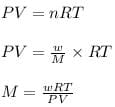where,
P = pressure of the gas
V = volume of the gas
T = temperature of the gas
n = number of moles of the gas
R = gas constant = 0.0821
Latm/moleK
M = molar mass of gas
w = mass of gas
Now put all the given values in the formula, we get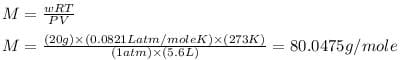Therefore, the molar mass of the gas is, 80.0475 g/mole

Chemistry Test 1 - Mole Concept, Atomic Structure, Bonding, Periodic Properties, General Organic Compounds - Question 2

### 5.6 litres of a gas at N.T.P. are found to have a mass of 11 g. The molecular mass of the gas is

Detailed Solution for Chemistry Test 1 - Mole Concept, Atomic Structure, Bonding, Periodic Properties, General Organic Compounds - Question 2
1 mole or molecular mass of the gas has volume 22.4 L
Given that:
11g of gas has volume 5.6 L

So molecular mass = (11/5.6)×22.4 = 44 u
Chemistry Test 1 - Mole Concept, Atomic Structure, Bonding, Periodic Properties, General Organic Compounds - Question 3

### A crystalline salt Na2SO4.xH2O on heating losses 55.9 per cent of its mass. The formula of crystalline salt is---

Detailed Solution for Chemistry Test 1 - Mole Concept, Atomic Structure, Bonding, Periodic Properties, General Organic Compounds - Question 3 Na2SO4.xH2O has weight =46+32+64+18x=142+18xg
on heating it will become anhydrous so, I.e. water will be evaporated.
55.9% of(142+18x)=18x=>79.378+10.062x=18x
=>7.938x=79.378=>x~10g
so Na2SO4.10H2O and this amount of water is called
water of crystallisation.
Chemistry Test 1 - Mole Concept, Atomic Structure, Bonding, Periodic Properties, General Organic Compounds - Question 4

Vapour density of a metal chloride is 66. Its oxide contains 53% metal. The atomic weight of the metal is

Detailed Solution for Chemistry Test 1 - Mole Concept, Atomic Structure, Bonding, Periodic Properties, General Organic Compounds - Question 4
Equivalent of metal = Equivalent of chloride

53/n = 47/8 , E = 9.02 , Atomic mass of metal = n X 9.02

Molecular mass of chloride = 2 X 66 = n X 9.02 + n X 35.5

on solving n = 3

Atomic mass of metal = 9.02 X 3 = 27.06
Chemistry Test 1 - Mole Concept, Atomic Structure, Bonding, Periodic Properties, General Organic Compounds - Question 5

When two aqueous solutions react together to give off solid product, this reaction is known as

Detailed Solution for Chemistry Test 1 - Mole Concept, Atomic Structure, Bonding, Periodic Properties, General Organic Compounds - Question 5
Chemical reactions:precipitation. The precipitate forms because the solid (AgCl) is insoluble in water. That is true for all precipitates - the solids are insoluble in aqueous solutions. Precipitation reaction occur all around us.
Chemistry Test 1 - Mole Concept, Atomic Structure, Bonding, Periodic Properties, General Organic Compounds - Question 6

At S.T.P. the density of CCl4 vapour in g/L will be nearest to

Detailed Solution for Chemistry Test 1 - Mole Concept, Atomic Structure, Bonding, Periodic Properties, General Organic Compounds - Question 6 Stp of mole of a gas = 22.4L 1mole --> 154g of CCl4 density=mass/volume =154/22.4 = 6.875
Chemistry Test 1 - Mole Concept, Atomic Structure, Bonding, Periodic Properties, General Organic Compounds - Question 7

Which of the following transitions of electrons in the hydrogen, atom will emit maximum energy ?

Detailed Solution for Chemistry Test 1 - Mole Concept, Atomic Structure, Bonding, Periodic Properties, General Organic Compounds - Question 7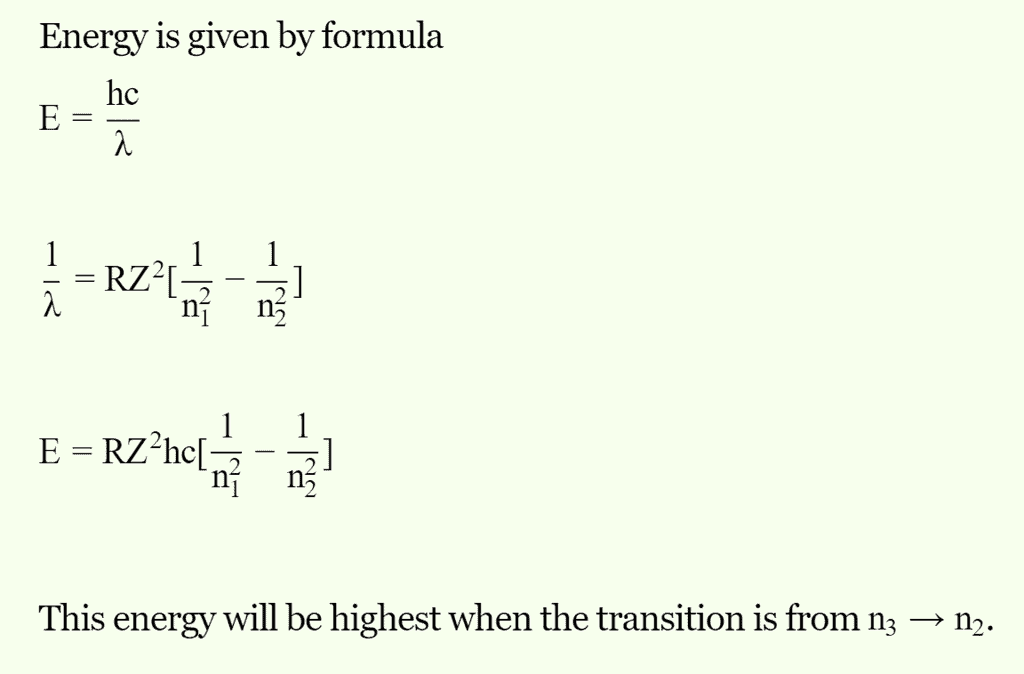Chemistry Test 1 - Mole Concept, Atomic Structure, Bonding, Periodic Properties, General Organic Compounds - Question 8

The uncertainty found from the uncertainty principle  (Δx.Δp = h/4 π) is

Detailed Solution for Chemistry Test 1 - Mole Concept, Atomic Structure, Bonding, Periodic Properties, General Organic Compounds - Question 8
Heisenberg uncertainty principle states that exact momentum and exact position of matter wave can't be determined simultaneously.
∆P.∆X>equal to h/4π
Chemistry Test 1 - Mole Concept, Atomic Structure, Bonding, Periodic Properties, General Organic Compounds - Question 9

The number of atoms of hydrogen in 2 moles of NH3

Detailed Solution for Chemistry Test 1 - Mole Concept, Atomic Structure, Bonding, Periodic Properties, General Organic Compounds - Question 9

Therefore 2 moles will have 1.204*1024 molecules of NH3.

There are 4 atoms in each molecule, so 2 moles will have 4*(1.204*1024) atoms

which is 4.816*1024 atoms.

Chemistry Test 1 - Mole Concept, Atomic Structure, Bonding, Periodic Properties, General Organic Compounds - Question 10

The spectrum of He is expected to be similar to that of

Detailed Solution for Chemistry Test 1 - Mole Concept, Atomic Structure, Bonding, Periodic Properties, General Organic Compounds - Question 10

The spectrum of an atom depends on the number of electrons present in it. Here, helium has two electrons, so the spectrum of Li+ (Z = 3) is similar to that of helium because both He and Li+ have two electrons.

Chemistry Test 1 - Mole Concept, Atomic Structure, Bonding, Periodic Properties, General Organic Compounds - Question 11

The electrons present in K-shell of the atom will differ in

Detailed Solution for Chemistry Test 1 - Mole Concept, Atomic Structure, Bonding, Periodic Properties, General Organic Compounds - Question 11
The electrons present in K-shell of the atom will differ in spin quantum number as the electrons will have opposite spins.
Chemistry Test 1 - Mole Concept, Atomic Structure, Bonding, Periodic Properties, General Organic Compounds - Question 12

Which of the following statements about quantum numbers is wrong?

Detailed Solution for Chemistry Test 1 - Mole Concept, Atomic Structure, Bonding, Periodic Properties, General Organic Compounds - Question 12

If the value of l=0, then electron lies in s-subshell which has spherical shape. So electron distribution is spherical.

Shape of orbital is given by azimuthal quantum number as it describes the subshell.

Magnetic quantum number determines the shift of atomic orbital due to external magnetic field i.e., zeeman effect.

Spin quantum number describes the spin of electron in particular orbital. Orientations of electron cloud is specified of a magnetic quantum number.

So B option is incorrect.

Chemistry Test 1 - Mole Concept, Atomic Structure, Bonding, Periodic Properties, General Organic Compounds - Question 13

Out of the two compounds shown below, the vapour pressing of B at a particular temperature is expected to be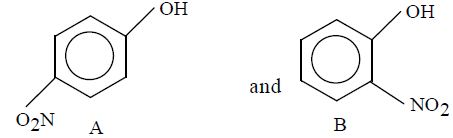Detailed Solution for Chemistry Test 1 - Mole Concept, Atomic Structure, Bonding, Periodic Properties, General Organic Compounds - Question 13

Because of intramolecular hydrogen bonding in (B), i.e., ortho nitrophenol, the vapour pressure of ortho nitrophenol is expected to be higher than paranitrophenol.

Therefore, the correct option is A.

Chemistry Test 1 - Mole Concept, Atomic Structure, Bonding, Periodic Properties, General Organic Compounds - Question 14

In which of the following molecules, the central atom does not use sP3-hybrid orbitals in its bonding ?

Detailed Solution for Chemistry Test 1 - Mole Concept, Atomic Structure, Bonding, Periodic Properties, General Organic Compounds - Question 14

In the given options, BeF3−​, Be forms three sigma bonds with three flourines.

So, hybridisation of Be becomes sp2.

Hence, the correct answer is option A.

Chemistry Test 1 - Mole Concept, Atomic Structure, Bonding, Periodic Properties, General Organic Compounds - Question 15

The correct increasing bond angle among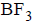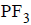and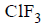Detailed Solution for Chemistry Test 1 - Mole Concept, Atomic Structure, Bonding, Periodic Properties, General Organic Compounds - Question 15

BF3​is trigonal planar which makes the angle as 120 degree.PF3​ is trigonal bipyramidal making the angle slightly less than 109 degrees ClF3​ is T-shaped making the least angle.

Chemistry Test 1 - Mole Concept, Atomic Structure, Bonding, Periodic Properties, General Organic Compounds - Question 16
Calculated bond order in the superoxide (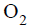) ion is
Detailed Solution for Chemistry Test 1 - Mole Concept, Atomic Structure, Bonding, Periodic Properties, General Organic Compounds - Question 16 O2^- (superoxide) = 13 valence e⁻. = σs(2 e⁻) σs*(2e⁻) σp(2e⁻) πp(4e⁻) πp*(3e⁻) σp*(0) . bond order = ½[ σs(2e⁻) σp(2e⁻) πp(4 e⁻) - σs*(2e⁻) πp*(3e⁻) ]. = ½[8-5] = 1.5
Chemistry Test 1 - Mole Concept, Atomic Structure, Bonding, Periodic Properties, General Organic Compounds - Question 17

The ionic species having largest size is

Detailed Solution for Chemistry Test 1 - Mole Concept, Atomic Structure, Bonding, Periodic Properties, General Organic Compounds - Question 17

Li+ ion being smallest in size is heavily hydrated and thus Li+(aq) is largest in size.

Chemistry Test 1 - Mole Concept, Atomic Structure, Bonding, Periodic Properties, General Organic Compounds - Question 18

From which of the following species it is easiest to remove one electron ?

Detailed Solution for Chemistry Test 1 - Mole Concept, Atomic Structure, Bonding, Periodic Properties, General Organic Compounds - Question 18

Energy is released when an electron is added to the atom. Hence 1st electron affinity (EA1​) is always taken as negative. The addition of second electron to the negativity charged ions is opposed by Coulombic repulsion, hence energy has to be supplied for the addition of second electron. Hence (EA2​) is taken as positive.

Addition of an electron to F− and O− ions will involve repulsions, hence their (EA2​) will be positive.
Due to stable fully filled configuration of Na+ (1s2 2s2 2p6) it has lower value of electron affinity than O. Hence O has highest electron affinity.

Chemistry Test 1 - Mole Concept, Atomic Structure, Bonding, Periodic Properties, General Organic Compounds - Question 19
With respect to chlorine, hydrogen will be
Detailed Solution for Chemistry Test 1 - Mole Concept, Atomic Structure, Bonding, Periodic Properties, General Organic Compounds - Question 19
With respect to Chlorine, hydrogen will be electropositive.

Reason:

Chlorine being a strong electronegative element tends to force hydrogen to lose electron and hydrogen acquire electropositive character so that chlorine can complete its octet.

Chemistry Test 1 - Mole Concept, Atomic Structure, Bonding, Periodic Properties, General Organic Compounds - Question 20

Which element has the greatest tendency to lose electrons ?

Detailed Solution for Chemistry Test 1 - Mole Concept, Atomic Structure, Bonding, Periodic Properties, General Organic Compounds - Question 20 Fe has high electronegativity. it is a metal and it has proper to lose electron
*Answer can only contain numeric values
Chemistry Test 1 - Mole Concept, Atomic Structure, Bonding, Periodic Properties, General Organic Compounds - Question 21

0.01 mole of iodoform (CHI3) reacts with Ag to produce a gas whose volume at NTP in ml is :-
2CHI3 + 6Ag —→ 6AgI(s) + C2H2(g)

Detailed Solution for Chemistry Test 1 - Mole Concept, Atomic Structure, Bonding, Periodic Properties, General Organic Compounds - Question 21

mol of C2H2 = 1/2 × mol of CHI3
= 1/2 × 0.01 = 0.005
volume at N.T.P. = 0.005 × 22400 = 112 ml

*Answer can only contain numeric values
Chemistry Test 1 - Mole Concept, Atomic Structure, Bonding, Periodic Properties, General Organic Compounds - Question 22

Sum of σ and π bonds are present in CH3COOH?

Detailed Solution for Chemistry Test 1 - Mole Concept, Atomic Structure, Bonding, Periodic Properties, General Organic Compounds - Question 22

Single bond is σ bond and double bond contains 1σ and 1 π bond. Therefore, it contains 7 σ and 1 π bond. Hence, total is 8

*Answer can only contain numeric values
Chemistry Test 1 - Mole Concept, Atomic Structure, Bonding, Periodic Properties, General Organic Compounds - Question 23

How many maximum electrons can be accomodate in 7th shell of element X?

Detailed Solution for Chemistry Test 1 - Mole Concept, Atomic Structure, Bonding, Periodic Properties, General Organic Compounds - Question 23

Maximum number of electron in a shell
= 2n2
= 2(7)2
= 98

*Answer can only contain numeric values
Chemistry Test 1 - Mole Concept, Atomic Structure, Bonding, Periodic Properties, General Organic Compounds - Question 24

How many groups show – I effect?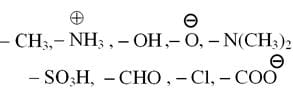Detailed Solution for Chemistry Test 1 - Mole Concept, Atomic Structure, Bonding, Periodic Properties, General Organic Compounds - Question 24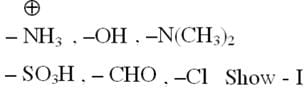*Answer can only contain numeric values
Chemistry Test 1 - Mole Concept, Atomic Structure, Bonding, Periodic Properties, General Organic Compounds - Question 25

Number of lone pair present in P4O6.

Detailed Solution for Chemistry Test 1 - Mole Concept, Atomic Structure, Bonding, Periodic Properties, General Organic Compounds - Question 25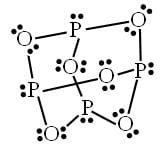## JEE Main & Advanced Mock Test Series

2 videos|326 docs|203 tests
 Use Code STAYHOME200 and get INR 200 additional OFF Use Coupon Code
Information about Chemistry Test 1 - Mole Concept, Atomic Structure, Bonding, Periodic Properties, General Organic Compounds Page
In this test you can find the Exam questions for Chemistry Test 1 - Mole Concept, Atomic Structure, Bonding, Periodic Properties, General Organic Compounds solved & explained in the simplest way possible. Besides giving Questions and answers for Chemistry Test 1 - Mole Concept, Atomic Structure, Bonding, Periodic Properties, General Organic Compounds, EduRev gives you an ample number of Online tests for practice

## JEE Main & Advanced Mock Test Series

2 videos|326 docs|203 tests